# 时间序列的7种预测模型

### 背景

from statsmodels.tsa.api import ExponentialSmoothing, \
SimpleExpSmoothing, Holt

import statsmodels.api as sm


### 1. 朴素估计

y ^ t + 1 = y t \hat{y}_{\mathrm{t}+1}=\mathrm{y}_{\mathrm{t}}

dd= np.asarray(train.Count)
y_hat = test.copy()
y_hat['naive'] = dd[len(dd)-1]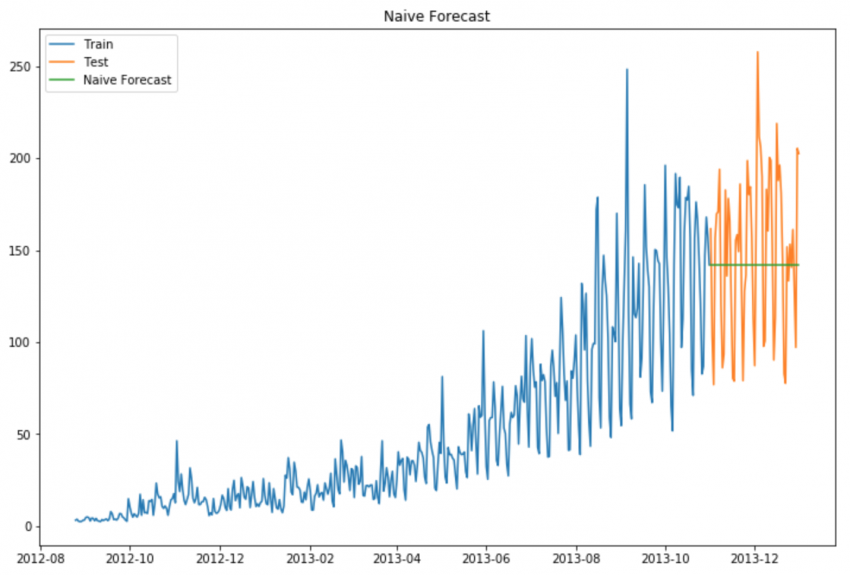### 2. 简单平均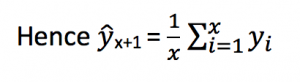y_hat_avg = test.copy()
y_hat_avg['avg_forecast'] = train['Count'].mean()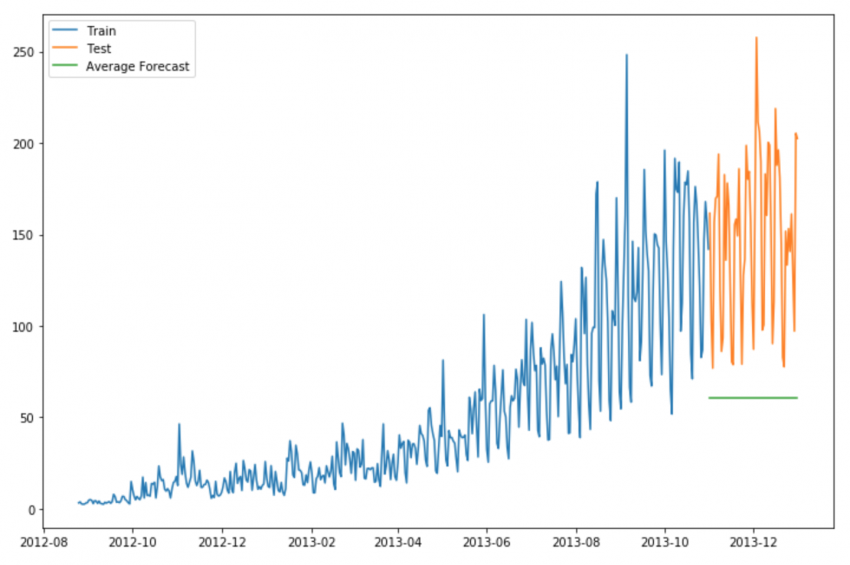### 4. 滑动窗平均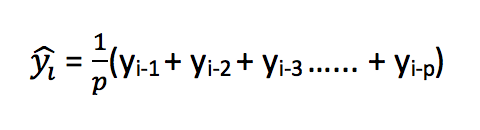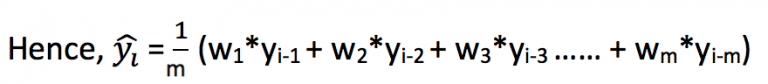y_hat_avg = test.copy()
y_hat_avg['moving_avg_forecast'] = train['Count'].rolling(60).mean().iloc[-1]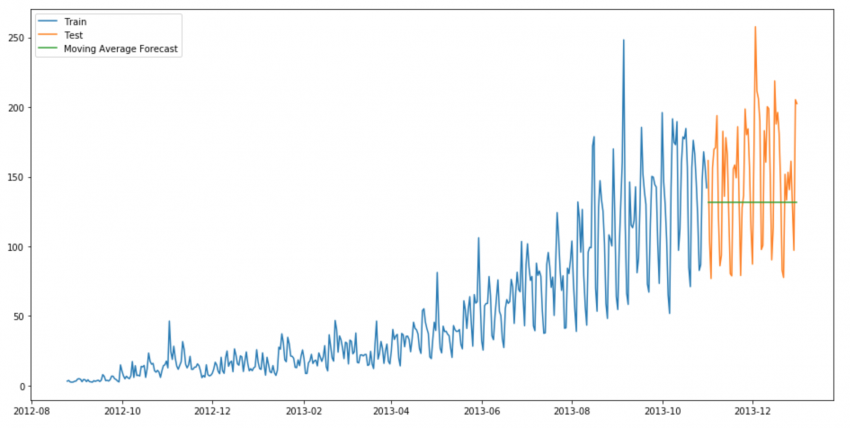### 5. 简单指数平滑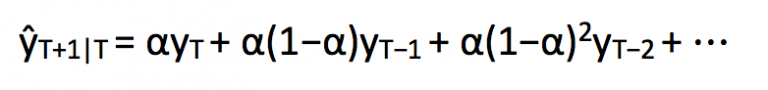where 0≤ α ≤1 是平滑参数.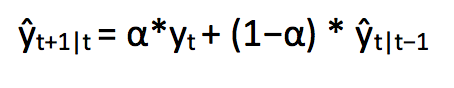from statsmodels.tsa.api import ExponentialSmoothing, \
SimpleExpSmoothing, Holt
y_hat_avg = test.copy()
fit2 = SimpleExpSmoothing(np.asarray(train['Count'])).fit(
smoothing_level=0.6,optimized=False)
y_hat_avg['SES'] = fit2.forecast(len(test))


### 5 Holt’s线性趋势方法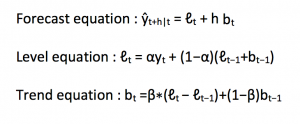import statsmodels.api as sm
sm.tsa.seasonal_decompose(train.Count).plot()


### 7 Holt-winters 方法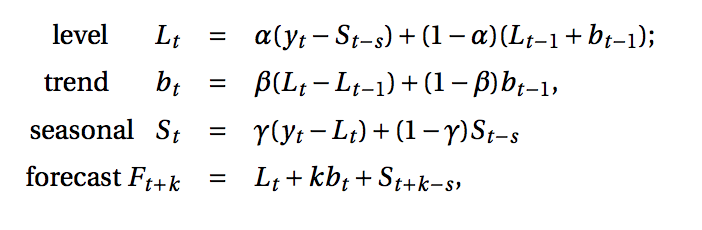1.数据是呈递增、递减趋势的；
2.数据服从一个周期变化。

y_hat_avg = test.copy()
fit1 = ExponentialSmoothing(np.asarray(train['Count']) ,
y_hat_avg['Holt_Winter'] = fit1.forecast(len(test))


### 8 Arima方法

ARIMA模型（Autoregressive Integrated Moving Average model）整合移动平均自回归模型。

ARIMA（p，d，q）模型：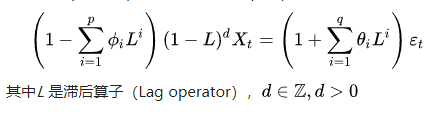ARIMA(p, d, q) 由三个部分组成：

• AR§：AR是autoregressive的缩写，表示自回归模型，含义是当前时间点的值等于过去若干个时间点的值的回归——因为不依赖于别的解释变量，只依赖于自己过去的历史值，故称为自回归；如果依赖过去最近的p个历史值，称阶数为p，记为AR(p)模型。
• I(d)：I是integrated的缩写，含义是模型对时间序列进行了差分；因为时间序列分析要求平稳性，不平稳的序列需要通过一定手段转化为平稳序列，一般采用的手段是差分；d表示差分的阶数，t时刻的值减去t-1时刻的值，得到新的时间序列称为1阶差分序列；1阶差分序列的1阶差分序列称为2阶差分序列，以此类推；另外，还有一种特殊的差分是季节性差分S，即一些时间序列反应出一定的周期T，让t时刻的值减去t-T时刻的值得到季节性差分序列。
• MA(q)：MA是moving average的缩写，表示移动平均模型，含义是当前时间点的值等于过去若干个时间点的预测误差的回归；预测误差=模型预测值-真实值；如果序列依赖过去最近的q个历史预测误差值，称阶数为q，记为MA(q)模型。
y_hat_avg = test.copy()
fit1 = sm.tsa.statespace.SARIMAX(train.Count, order=(2, 1,
4),seasonal_order=(0,1,1,7)).fit()
y_hat_avg['SARIMA'] = fit1.predict(start="2013-11-1",
end="2013-12-31", dynamic=True)


### 9. PROPHET 方法

fbprophet的安装依赖Pystan.reference：

01-163万+
04-161万+01-089675
08-211万+
05-201万+
12-306761
04-222万+
11-101万+
06-053506
11-0318万+